Analog Electronics - 1

# Analog Electronics - 1

Test Description

## 10 Questions MCQ Test GATE ECE (Electronics) 2023 Mock Test Series | Analog Electronics - 1

Analog Electronics - 1 for Electronics and Communication Engineering (ECE) 2023 is part of GATE ECE (Electronics) 2023 Mock Test Series preparation. The Analog Electronics - 1 questions and answers have been prepared according to the Electronics and Communication Engineering (ECE) exam syllabus.The Analog Electronics - 1 MCQs are made for Electronics and Communication Engineering (ECE) 2023 Exam. Find important definitions, questions, notes, meanings, examples, exercises, MCQs and online tests for Analog Electronics - 1 below.
Solutions of Analog Electronics - 1 questions in English are available as part of our GATE ECE (Electronics) 2023 Mock Test Series for Electronics and Communication Engineering (ECE) & Analog Electronics - 1 solutions in Hindi for GATE ECE (Electronics) 2023 Mock Test Series course. Download more important topics, notes, lectures and mock test series for Electronics and Communication Engineering (ECE) Exam by signing up for free. Attempt Analog Electronics - 1 | 10 questions in 30 minutes | Mock test for Electronics and Communication Engineering (ECE) preparation | Free important questions MCQ to study GATE ECE (Electronics) 2023 Mock Test Series for Electronics and Communication Engineering (ECE) Exam | Download free PDF with solutions
 1 Crore+ students have signed up on EduRev. Have you?
Analog Electronics - 1 - Question 1

### A half-wave rectifier is used to charge a 12V battery through a resistance ‘R’. The input transformer is fed with 34 AC with turns ration 2 : 1. The conduction period of the diode is

Detailed Solution for Analog Electronics - 1 - Question 1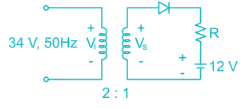Voltage at secondary winding of the coil is Vs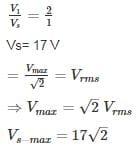Voltage across diode

Vp → Vs max

Vn → 12 V

For conduction:

Vp > Vn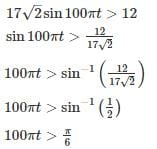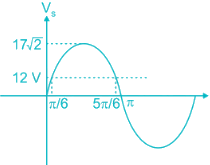Therefore the diode is conducting for the period.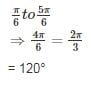*Answer can only contain numeric values
Analog Electronics - 1 - Question 2

### A full-wave rectifier circuit employs inductor filter at its output. If the source frequency is 50 Hz and the inductor value is 10 mH. Find the ripple factor for 100 Ω loads.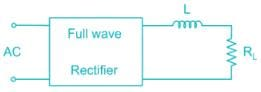Detailed Solution for Analog Electronics - 1 - Question 2

For full wave rectifier using inductor filter at output is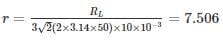*Answer can only contain numeric values
Analog Electronics - 1 - Question 3

### The voltage source VAB = 4 sin ωt is applied to the terminal A and B of the circuit shown In Fig. the diode is assumed to be ideal. The impedance by the circuit across the terminals A and B is ________ kΩ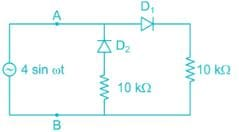Detailed Solution for Analog Electronics - 1 - Question 3

When,

D1 → ON; D2 → OFF: RAB = 10 kΩ

D2 → ON; D1 → OFF: RAB = 10 kΩ

∴ The impedance by the circuit across the terminals A and B  of 10 kΩ

Analog Electronics - 1 - Question 4

For the damping network shown below, resulting output for the applied Input will be…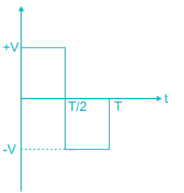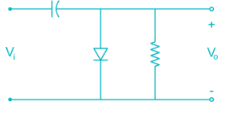Detailed Solution for Analog Electronics - 1 - Question 4

Clamping network “Clamp” a signal to different DC level.

During the Internal O → T/1 → Diode in the ON state during this internal output voltage is directly across the short circuit and Vo = 0 volts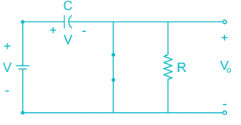When input switch to -V  state → diode OFF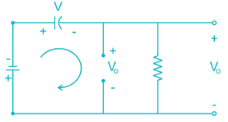Applying Kirchhoff’s voltage law around loop we get

-V -V -Vo = 0

Vo = -2V

So output wave form will be,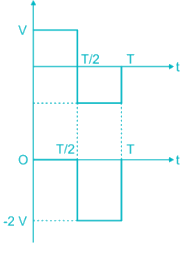*Answer can only contain numeric values
Analog Electronics - 1 - Question 5

Assuming the diode to be ideal, the current I in the circuit shown is ________ mA.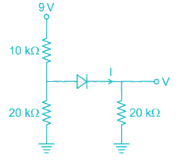Detailed Solution for Analog Electronics - 1 - Question 5

The above circuit can be redrawn as: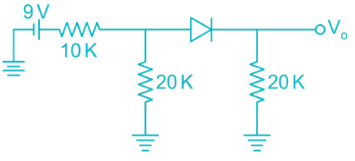Assume diode is off:

Voltage across 20 k resistor connected to left of diode is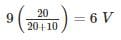This will turn on the diode

the equivalent circuit is now: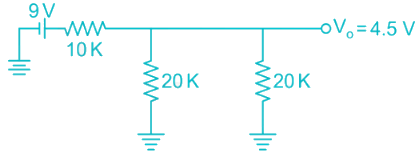Now the two 20k resistors are in parallel whose equivalent is 10k

this 10 k is in series with other 10k resistor

9V divides equally among them

V0 = 4.5V

Cuurent I = 4.5/20 mA

= 0.225 mA

Analog Electronics - 1 - Question 6

The correct option matching List - I to List - II is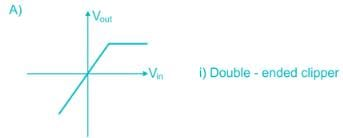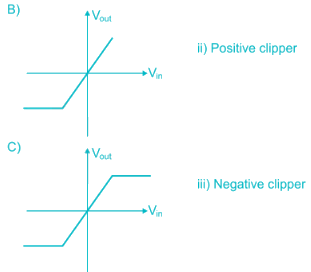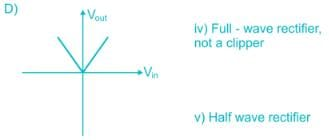Detailed Solution for Analog Electronics - 1 - Question 6

A) In graph ‘A’ the output follows input, but above certain positive input, output stays constant. The circuit is called positive clipper.

B) Similarly the graph ‘B’ is of negative clipper.

C) In this path positive and negative side are clipped, it is a double-ended clipper.

D) In this input output follows relation Vout = |Vin|. This is a full-wave rectifier circuit.

*Answer can only contain numeric values
Analog Electronics - 1 - Question 7

The figure shows a half wave rectifier with a load resistor of 50 Ohms. The input supply voltage is 230V(runs) at 50 Hz. A 10000 μF filter capacitor is added across the load resistor to reduce ripple. The percentage of ripple voltage at the output is ________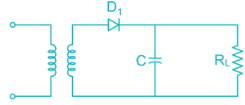Detailed Solution for Analog Electronics - 1 - Question 7

Due to filter capacitor the output voltage is given by waveform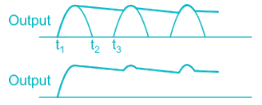Output DC voltage  Vavg = V= √2 × 230
= 325.3 V

The load current is given by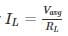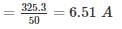This current discharges the capacitor to the load resistance during the interval (t2 to t3)

The time period of AC voltage.

= 20 ms (for 50 Hz)

Thus the charge supplied by the capacitor to the load resistance during this interval will be

∆Q = ILT = 6.51 × 20 × 10-3

= 0.1302 coulombs

Peak to peak ripple voltage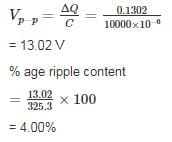Analog Electronics - 1 - Question 8

For the diode network shown below is input with voltage pulse Vi. If the knee voltage of the diode is Vk = 0.7 V then the output voltage pulse Vo is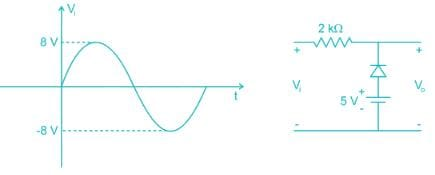Detailed Solution for Analog Electronics - 1 - Question 8

Given circuit can be written with ideal diode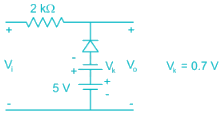Is input with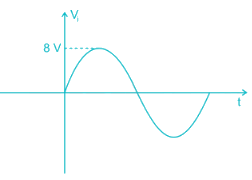During positive values of Vi < s – Vk i.e. Vi < 4.3 V the diode is in on-state, the diode can be taken as short circuit. The output voltage is simply be voltage across 5V voltage source.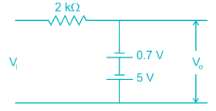⇒ Vo = s – 0.7 V

= 4.3 V

For Vi > 4.3 V the diode is in off-state the circuit become open circuit hence Vo = Vi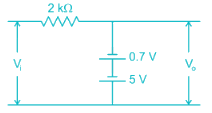The circuit is in on-state for entire negative cycle. Hence Vo = 4.3 V

The wave form of output voltage is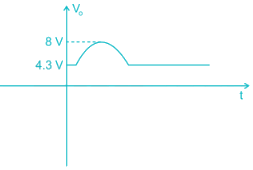*Answer can only contain numeric values
Analog Electronics - 1 - Question 9

In Fig. the Input Vis 100 Hz triangular wave having a peak to peak amplitude of 2 volts and an average value of 0 volts. Given that the diode is ideal, the average value of output Vo is ______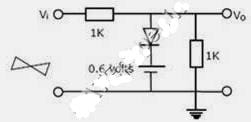Detailed Solution for Analog Electronics - 1 - Question 9

Time period = 1/f = 1/100 = 10msec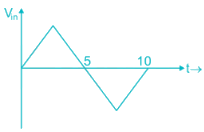1) During +Ve ½ cycle

Vin = + Ve diode is not forward bias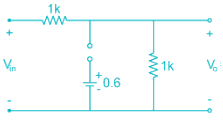2) During – Ve cycle

Vin = - Ve diode is Reverse Bias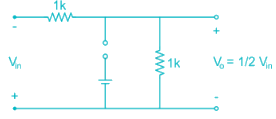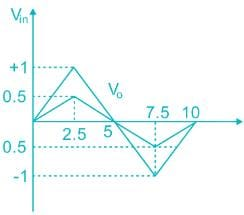Average value  = 0

*Answer can only contain numeric values
Analog Electronics - 1 - Question 10

In the circuit shown I is a dC current and Vi is a sinusoidal signal with peak amplitude 10 mV and a frequency 100 kHz. The value of I that will provide a phase shift of -45° at the output is ________. μA (Assume η = 1)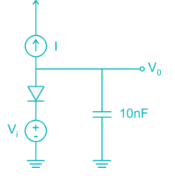Detailed Solution for Analog Electronics - 1 - Question 10

Opening the current source we get the AC small-signal circuit (η = 1)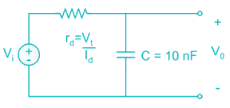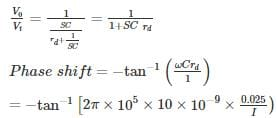I = 157 uA

## GATE ECE (Electronics) 2023 Mock Test Series

21 docs|263 tests
 Use Code STAYHOME200 and get INR 200 additional OFF Use Coupon Code
Information about Analog Electronics - 1 Page
In this test you can find the Exam questions for Analog Electronics - 1 solved & explained in the simplest way possible. Besides giving Questions and answers for Analog Electronics - 1, EduRev gives you an ample number of Online tests for practice

## GATE ECE (Electronics) 2023 Mock Test Series

21 docs|263 tests